## SQL Server 2008: Analysis Services

#### Course at a glance

Included in these subscriptions:

• Dev & IT Pro Video
• Dev & IT Pro Power Pack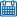Release date 9/24/2009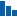Level Advanced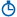Runtime 16h 19m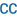Closed captioning N/A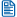Transcript N/A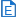eBooks / courseware Included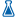Hands-on labs Included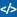Sample code Included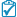Exams Included#### Course description

In this course, you will learn how to use Microsoft SQL Server 2008 Analysis Services (SSAS) to design and implement OnLine Analytical Processing (OLAP) cubes, cube storage and aggregation designs to support Business Intelligence (BI) solutions. This course includes concepts, procedures and practices based on real-world experience giving both the novice and experienced SQL Server 2008 developer the tools to build data cubes based upon measures and dimensions.

#### Learning Paths

This course will help you prepare for the following certification and exam:
MCTS: SQL Server 2008, Business Intelligence Development and Maintenance
70-448: TS: Microsoft SQL Server 2008, Business Intelligence Development and Maintenance

#### Meet the expertAnn Weber has been an author, instructor, and consultant for over 12 years. She is an expert in SQL Server and has her MCITP, MCSE, and MCT certifications. Ann works with all facets of SQL Server including administration, writing queries, development, SSAS, SSIS, and SSRS. Ann has developed several courses and other learning materials for SQL Server.

#### Module 1

##### What is MS BI (22:57)
• Introduction (02:37)
• What is MS BI (00:48)
• Defining Microsoft BI (00:55)
• Why Use OLAP (01:36)
• Understanding the UDM (01:08)
• Understanding the Cube (00:14)
• Dimensions and Measures (00:47)
• OLAP Schemas (01:47)
• Sample Databases (00:33)
• Deploying/Viewing Cube (00:21)
• Deploy a Cube with BIDS (00:33)
• Demo: SSMS (01:26)
• Demo: BIDS in VS (08:12)
• Demo:SSMS Object Explorer (01:23)
• Summary (00:31)
##### SSAS Client Overview (13:18)
• Introduction (00:23)
• Viewing a Cube in Excel (01:06)
• Cubes in Reporting Services (01:16)
• Demo: Excel in RS (07:57)
• Demo: RS in SQL 08 (02:17)
• Summary (00:17)
##### OLAP Modeling (27:03)
• Introduction (00:58)
• Before Creating a Cube (01:16)
• Selecting a Modeling Tool (00:55)
• Understanding OLAP Modeling (01:55)
• Required Components (00:46)
• Star Schema Models (01:53)
• Dimensional Modeling (02:55)
• Demo: Visio Modeling (02:58)
• Understanding Cube Modeling (02:12)
• Dimension Types (03:14)
• Modeling Fact Tables (01:38)
• Demo: Visio Model Cube (03:28)
• Summary (01:03)

#### Module 2

##### Modeling in BIDS (27:04)
• Introduction (00:39)
• Understanding BIDS Interface (01:52)
• Modeling with BIDS (01:02)
• Demo: Modeling in BIDS (02:33)
• Demo: Cube (05:30)
• Demo: Create a new Database (13:05)
• Preparing Data (01:30)
• Summary (00:50)
##### Using BIDS (13:57)
• Introduction (01:09)
• Understanding BIDS (01:15)
• Offline vs. Online Mode (01:11)
• Demo: BIDS (01:48)
• Creating DataSources (01:31)
• Creating DataSource Views (02:22)
• Demo: DataSource (03:01)
• Demo: DataSource View Designer (01:10)
• Summary (00:27)
##### Creating a Cube (36:46)
• Introduction (00:57)
• Creating a Cube (00:49)
• Refine the Cube (01:12)
• Refining Dimensions (02:44)
• Refining Measures (00:44)
• Define Dimension Usage (01:39)
• Demo:Cube Designer (08:02)
• Demo:Dimension Designer (09:12)
• Demo:Dimension Wizard (05:18)
• Demo:Dim - Measure Groups (02:05)
• Demo:Deploy Cube (03:36)
• Summary (00:21)

#### Module 3

##### Enhancing a Cube (35:23)
• Introduction (01:47)
• Attribute Relationships (02:31)
• Demo: Relationships (05:25)
• Creating KPIs (01:55)
• Defining a KPI (02:03)
• Customizing KPI Samples (00:52)
• Demo: KPIs in a Cube (08:44)
• Demo: Growth KPI (02:31)
• Creating Perspectives (02:16)
• Demo: Perspectives (05:31)
• Summary (01:43)
##### Translating An Action (32:16)
• Introduction (01:11)
• Creating Translations (01:33)
• Demo: Translations (06:25)
• Localizing Measure Values (01:23)
• Currency Localization (00:45)
• Config Currency Localization (02:45)
• Demo: Currency Conversions (03:34)
• Actions (02:13)
• Demo: Actions (07:58)
• Demo: Action Creation (03:34)
• Summary (00:49)

#### Module 4

• Introduction (00:44)
• Multiple Fact Tables (02:23)
• Cube Multiple Fact Tables (02:39)
• Modeling Separate Cubes (01:02)
• Dimension Usage Config (00:39)
• Snowflake Dimensions (00:41)
• Degenerate Dimensions (00:59)
• Parent Child Dimensions (01:21)
• Many to Many Dimensions (01:09)
• Role Playing Dimensions (01:02)
• Writeback Dimensions (01:01)
• Demo: Dimension Types (04:22)
• Changing Dimensions (05:13)
• Summary (00:41)
##### BI Wizard- Advanced Program (32:30)
• Introduction (00:56)
• Using the BI Wizard (00:55)
• Wizard Dimension Options (03:07)
• Demo: BI Wizard (09:44)
• BI Wizard Cube Options (01:49)
• Demo: Cube Options (08:57)
• Demo: Browse Cube (05:55)
• Summary (01:03)

#### Module 5

• Introduction (00:31)
• Cube Dimension Properties (02:09)
• Dimension Properties (02:59)
• Demo:Cube/Dim Properties (06:51)
• Hierarchy Properties (01:07)
• Attribute Properties (00:58)
• Demo: Hierarchy/Attributes (21:58)
• Cube Properties (01:28)
• Measure Group Properties (03:19)
• Demo: Cube/Measure (04:34)
• Summary (01:38)
##### Storage And Aggregations (36:34)
• Introduction (00:46)
• Basic Storage- SSAS Databases (01:08)
• SSAS Data and Metadata (00:45)
• Demo: XMLA (09:25)
• Three Storage Modes (01:00)
• MOLAP (01:36)
• ROLAP (01:26)
• HOLAP (01:15)
• Overview of Aggregations (01:35)
• Defining Aggregations (01:56)
• Viewing Aggregation Designs (00:29)
• Demo: Aggregation Designs (13:26)
• Summary (00:49)

#### Module 6

• Introduction (00:40)
• Customizing Aggregations (01:38)
• Usage-Based Optimization (02:49)
• Demo: Usage-Based Wizard (14:46)
• Using SQL Profiler (02:46)
• Demo: SQL Profiler (11:15)
• Summary (00:45)
• Introduction (00:47)
• Storage Mode Settings (04:27)
• ROLAP Dimensions (01:35)
• Demo: Storage Modes (03:14)
• Implementing Proactive Caching (01:32)
• Defining Proactive Caching (03:08)
• Partition Locations (01:08)
• Using Partitions-Where? (00:51)
• Demo: Partitions in a Cube (08:47)
• General Processing Options (02:32)
• Measure Groups+Partitions (00:38)
• Dimension Processing Options (00:36)
• Data Mining Processing Options (00:36)
• Processing Settings (01:42)
• Demo: Processing in BIDs (06:20)
• Summary (01:06)

#### Module 7

##### MDX Overview (28:26)
• Introduction (00:45)
• Understanding MDX (00:50)
• Using MDX in BIDS (01:17)
• MDX Queries (00:37)
• MDX Expressions (00:48)
• MDX Structure Names (03:30)
• Demo: MDX Structure (05:14)
• MDX Syntax Rules (01:49)
• Writing MDX Query (01:20)
• Demo: Writing MDX Queries (11:24)
• Summary (00:46)
##### MDX Queries (20:08)
• Introduction (00:49)
• Members, Tuples, and Sets (03:49)
• Demo: Tuples and Sets (06:31)
• Familial Relationships (01:26)
• Demo: Navigtional Functions (05:36)
• Summary (00:38)
##### MDX Functions (24:40)
• Introduction (00:34)
• MDX Functions & Keywords (00:55)
• Demo: MDX Functions (03:32)
• Functions & Keywords (cont) (02:39)
• Demo: Functions (cont) (04:08)
• More Functions (02:57)
• Functions added to SSAS 05 (02:45)
• Demo: More Functions (06:27)
• Summary (00:40)

#### Module 8

##### Calculated Members (30:41)
• Introduction (00:27)
• Using MDX Expressions in BIDS (01:00)
• Demo: MDX in BIDS (02:47)
• Working with Calculations (00:12)
• The Calculations Subtab (01:53)
• Demo: Calculations Subtab (04:13)
• Examine Calculated Members (01:23)
• Why Use Calculated Members (01:19)
• Defining Calculated Members (02:16)
• Demo: Calculated Members (14:39)
• Summary (00:27)
##### Script Commands & .NET (30:30)
• Introduction (00:32)
• Demo: Script Commands (06:01)
• Demo: Break Points (02:53)
• Understand Named Sets (01:18)
• Creating Named Sets (01:56)
• Demo: Named Sets (09:09)
• Why Assemblies? (00:46)
• Demo: Register a .DLL (02:48)
• Summary (00:27)
##### Data Mining Overview (15:30)
• Introduction (00:50)
• What is Data Mining (00:48)
• Data Mining Terminology (01:44)
• Demo: Mining Model (01:34)
• Algorithm Classifications (05:02)
• Implementing Data Mining (02:35)
• Summary (00:27)
##### Implementing Data Mining (20:05)
• Introduction (00:33)
• Mining Algorithms (04:43)
• Demo:Mining Models (03:32)
• DM Algorithms 1 (02:18)
• DM Algorithms 2 (04:13)
• DM Algorithms 3 (02:17)
• DM Algorithms 4 (02:08)
• Summary (00:18)

#### Module 9

##### Creating Mining Structures (22:17)
• Introduction (00:26)
• Creating Mining Structures (01:46)
• Demo: Data Mining Wizard (05:30)
• Content Types (04:41)
• Data Types (01:04)
• Data Types (cont) (00:43)
• Finishing the Wizard (01:20)
• Demo: Wizard from Cube (06:17)
• Summary (00:26)
##### Data Mining Designer (34:50)
• Introduction (00:41)
• Mining Structure Subtab (02:07)
• Mining Models Subtab (02:42)
• Demo:Structure+Models Subtabs (06:17)
• Mining Model Viewers (04:26)
• Demo: Model Viewers (18:10)
• Summary (00:25)
##### Validating Mining Models (42:24)
• Introduction (00:53)
• Mining Accuracy Charts (04:04)
• Demo: Accuracy Charts (09:21)
• Cross Validation (02:17)
• Demo: Cross Validation (05:04)
• Mining Prediction Viewers (01:08)
• Working with the DMX (01:08)
• Demo: Query in BIDS (05:28)
• Demo: Query in SSMS (11:18)
• Mining Structure Processing (01:06)
• Summary (00:30)

#### Module 10

##### Securing SSAS (32:36)
• Introduction (00:28)
• Implementing Security (01:21)
• Reducing the Attack Surface (01:23)
• Data Connection Methods (02:12)
• Demo: Configure Security (07:03)
• Implement Database Roles (01:49)
• Securing Dimensions (02:21)
• Security Considerations (01:31)
• Demo: Roles + Permissions (12:21)
• Summary (00:25)
##### Scripting SSAS (19:49)
• Introduction (00:48)
• Implement XMLA Scripts (02:15)
• Demo: XMLA Script Templates (03:01)
• XMLA Schema Rowsets (01:43)
• OLE DB Schema Rowsets (00:50)
• OLE DB OLAP Rowsets (01:17)
• Data Mining Schema Rowsets (00:40)
• Demo: Data Mining Rowsets (07:20)
• Demo: Schema Rowsets (01:37)
• Summary (00:14)
##### Managing SSAS (34:57)
• Introduction (00:36)
• Deploy and Synchronizing (00:47)
• Deploying Using BIDS (02:22)
• Deployment Wizard (02:15)
• Database Synchronization (01:35)
• Demo:Database Deployment (10:01)
• Understand Backup/Restore (01:03)
• What to Backup (00:43)
• SSAS Database Backups (01:54)
• Demo: Backup and Restore (08:33)
• Demo: Backup to a Job (04:43)
• Summary (00:21)

#### Module 11

##### SSIS with SSAS (19:32)
• Introduction (00:24)
• Using SSIS with SSAS (00:45)
• SSIS to Process Partitions (01:19)
• Other SSIS AS Tasks (01:23)
• Demo: SSIS tasks in AS (13:22)
• Summary (00:27)
##### Availability and Scalability (15:29)
• Introduction (00:34)
• Availability and Scalability (00:43)
• Understanding Clustering (01:33)
• Scale-up Solutions (01:18)
• Demo: Scalability Properties (05:38)
• Summary (00:29)
##### Optimizing Performance (31:38)
• Introduction (00:26)
• Optimization Overview (01:30)
• Design Efficiently (03:36)
• Retrieve Data Efficiently (01:40)
• Aggregate Appropriately (01:00)
• Optimize Attributes (00:59)
• Monitor Intelligently (01:25)
• Demo: Perfmon and Profiler (17:13)
• Query Efficiently (02:33)
• Summary (01:10)
##### Excel 2007 (30:07)
• Introduction (00:39)
• Excel 2007 (00:37)
• Managing Connections in Excel (00:31)
• Excel PivotTable Reports (01:38)
• Demo: Excel Connections (05:20)
• Demo: Excel PivotTable (08:01)
• Excel PivotChart Reports (01:06)
• Demo: PivotCharts (04:56)
• OLAP Tools (00:47)
• Demo: OLAP Tools (06:08)
• Summary (00:19)

#### Module 12

##### Data Mining Add Ins (40:40)
• Introduction (00:38)
• Excel as a Data Mining Client (01:59)
• Data Mining Add-ins Interface (01:04)
• Demo: DataMining Ribbon (00:59)
• Data Preparation (01:19)
• Demo: Data Preparation (07:28)
• Data Modeling (00:24)
• Accuracy and Validation (00:50)
• Demo: Data Modeling (06:55)
• Demo: Accuracy and Validation (05:50)
• Model Usage (00:33)
• Management, Connections (00:48)
• Demo:Management (08:11)
• Data Mining Template Visio (00:44)
• Demo: Data Mining Template (02:34)
• Summary (00:17)
##### SSRS with SSAS (24:55)
• Introduction (00:42)
• Demo: SSRS (03:52)
• SSRS-Designing Reports (01:11)
• SSRS and SSAS (01:12)
• Demo: SSRS with SSAS (16:01)
• Summary (00:15)
##### SharePoint Integration (18:10)
• Introduction (00:24)